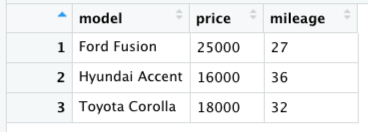# 3.8: Data Frames

$$\newcommand{\vecs}{\overset { \rightharpoonup} {\mathbf{#1}} }$$ $$\newcommand{\vecd}{\overset{-\!-\!\rightharpoonup}{\vphantom{a}\smash {#1}}}$$$$\newcommand{\id}{\mathrm{id}}$$ $$\newcommand{\Span}{\mathrm{span}}$$ $$\newcommand{\kernel}{\mathrm{null}\,}$$ $$\newcommand{\range}{\mathrm{range}\,}$$ $$\newcommand{\RealPart}{\mathrm{Re}}$$ $$\newcommand{\ImaginaryPart}{\mathrm{Im}}$$ $$\newcommand{\Argument}{\mathrm{Arg}}$$ $$\newcommand{\norm}{\| #1 \|}$$ $$\newcommand{\inner}{\langle #1, #2 \rangle}$$ $$\newcommand{\Span}{\mathrm{span}}$$ $$\newcommand{\id}{\mathrm{id}}$$ $$\newcommand{\Span}{\mathrm{span}}$$ $$\newcommand{\kernel}{\mathrm{null}\,}$$ $$\newcommand{\range}{\mathrm{range}\,}$$ $$\newcommand{\RealPart}{\mathrm{Re}}$$ $$\newcommand{\ImaginaryPart}{\mathrm{Im}}$$ $$\newcommand{\Argument}{\mathrm{Arg}}$$ $$\newcommand{\norm}{\| #1 \|}$$ $$\newcommand{\inner}{\langle #1, #2 \rangle}$$ $$\newcommand{\Span}{\mathrm{span}}$$$$\newcommand{\AA}{\unicode[.8,0]{x212B}}$$

Often in a dataset we will have a number of different variables that we want to work with. Instead of having a different named variable that stores each one, it is often useful to combine all of the separate variables into a single package, which is referred to as a data frame.

If you are familiar with a spreadsheet (say from Microsoft Excel) then you already have a basic understanding of a data frame.
Let’s say that we have values of price and mileage for three different types of cars. We could start by creating a variable for each one, making sure that the three cars are in the same order for each of the variables:

car_model <- c("Ford Fusion", "Hyundai Accent", "Toyota Corolla")
car_price <- c(25000, 16000, 18000)
car_mileage <- c(27, 36, 32)


We can then combine these into a single data frame, using the data.frame() function. I like to use "_df" in the names of data frames just to make clear that it’s a data frame, so we will call this one “cars_df”:

cars_df <- data.frame(model=car_model, price=car_price, mileage=car_mileage)


We can view the data frame by using the View() function:

View(cars_df)

Which will present a view of the data frame much like a spreadsheet, as shown in Figure 2.1:Figure 2.1: A view of the cars data frame generated by the View() function.

Each of the columns in the data frame contains one of the variables, with the name that we gave it when we created the data frame. We can access each of those columns using the $ operator. For example, if we wanted to access the mileage variable, we would combine the name of the data frame with the name of the variable as follows: > cars_df$mileage
 27 36 32


This is just like any other vector, in that we can refer to its individual values using square brackets as we did with regular vectors:

> cars_df\$mileage
 32


In some of the examples in the book, you will see something called a tibble; this is basically a souped-up version of a data frame, and can be treated mostly in the same way.

This page titled 3.8: Data Frames is shared under a CC BY-NC 2.0 license and was authored, remixed, and/or curated by Russell A. Poldrack via source content that was edited to the style and standards of the LibreTexts platform; a detailed edit history is available upon request.